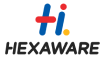New update is available. Click here to update.

# Sum Of Infinite Array

Medium0/80
35 mins
70 %3317 upvotes+1 more

## Problem Statement

#### Note :

``````The value of the sum can be very large, return the answer as modulus 10^9+7.
``````
Detailed explanation ( Input/output format, Notes, Images )
##### Constraints :
``````1 <= T <= 100
1 <= N <= 10^4
1 <= A[i] <= 10^9
1 <= Q <= 10^4
1 <= L <= R <= 10^18

Time Limit: 1sec
``````
##### Sample Input 1 :
``````1
3
1 2 3
2
1 3
1 5
``````
##### Sample Output 1 :
``````6 9
``````
##### Explanation to Sample Input 1 :
``````For the first test case, the given array A is [1,2,3] therefore the infinite array “B” will be [1,2,3,1,2,3,1,2,3,.......]. So the answer for the given first query is 6 because the sum of the subarray from index 1 to 3 of infinite array “B” i.e. (B+B+B) is 6.

For the given second query is 9 because the sum of the subarray from index 1 to 5 of array “B” .ie (B+B+B+B+B) is 9.
``````
##### Sample Input 2 :
``````1
4
5 2 6 9
3
1 5
10 13
7 11
``````
##### Sample Output 2 :
``````27 22 28
``````AutocompleteConsole